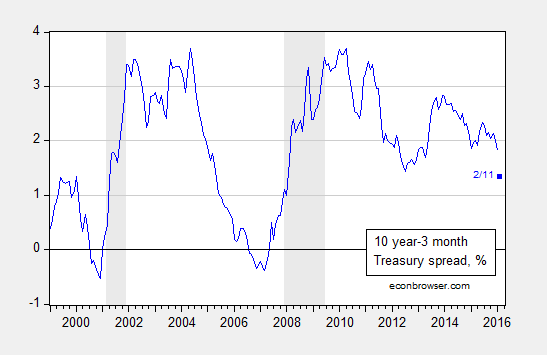# Yield Curve, February 11th

Given worries regarding an imminent slowdown in the wake of the stock market decline, it’s of interest to see what the term premium is signalling.Figure 1: Ten year minus three month Treasury yield spread, % (blue). February observation is for 11 February. NBER defined recession dates shaded gray. Source: FRB via FRED, NBER, and author’s calculations.

Estimating a standard probit model for recessions based on one year lagged term spread over the 1986-2015 period (encompassing the “Great Moderation”) yields:

Prob(recession=1)t = -0.142 – 0.871×spreadt-12 + ut

McFadden-R2 = 0.30. NObs = 360.

[figures edited 2/13 12:45am, to add 2015M02-2015M12 observations]
Where bold denotes significance at 1% msl, using Huber-White standard errors. Using a 20% threshold, the model predicts recession dates correctly 78% of the time, and no recession dates 85% of the time.

The spread on 11 February was 1.35 percentage points. Putting this into the estimated equation yields a value of -1.32, implying a 9.3% probability of a recession within the next year. This is a slightly different probability than that reported in this post due to the flattening of the yield curve since January 11th, and a slightly different sample period (the previous used 1970-2013).

## 9 thoughts on “Yield Curve, February 11th”

1. AS

Professor Chinn,
Are you using zeros or NA for the NBER recession index past the June 2014 last entry by NBER of June 2014? I am trying to match your data am having trouble getting the same results as you did. My coefficient on the spread is close at -0.84, but my constant is different at 0-.26, using data from 1986m01 to 2015m12 and a T10Y3m(-12) entry.

1. Menzie Chinn Post author

AS: I realize now that I had “NA” entries for 2015M03-2015M12. I have set those entries to zero, and re-run the regressions. The new results (only very slightly altered) are now reported. Thanks for catching that.

Also note that when the sample is 1986M01-2015M12, then this includes yield spread from 1985M12.

1. AS

Professor Chinn,
Using T10Y3M from 1985m12 to 2015m12 shows an output of 361 observations. Also, using the Probit model of: “Recession c T10Y3m(-12)” gives me an output of Prob(recession=1)t = -0.262 – 0.850 x T10Y3M . Evaluating the equation at the February spread of 1.35 percentage points, with recession equal to USRECM and using Norm S Dist I calculate a probability of recession of 7.9%. Thus, I seem to have gone astray.

1. Menzie Chinn Post author

AS: In EViews set smpl to 1986M01-2015M12. Since the spread is lagged 12 months, then you should end up with 360 observations. In addition, I’m using a manually generated spread, subtracting TB3MS from GS10. Not sure if you are using the same. Then we should get to similar results.

1. AS

Professor Chinn,
When I switched to using the secondary market 3 month TB data series, my calculations came close to yours at a forecast of 9.5%. Hopefully, I am not making a simple mistake. Perhaps different machines can have slightly different results for non linear calculations?

A learning question about the use of the secondary market data series, rather than using the FRED series of “T10Y3M”: Why use the secondary market data instead of the FRED series?
Thanks

2. Menzie Chinn Post author

AS: At this short of a horizon, I think the actually reported figures are better than (an estimated) constant maturity. At the ten year horizon, the difference between the on-the-run 10 year and 10 year constant maturity can be more substantial.

2. Kdavies

Professor Chinn, thank you for an interesting post – in using the yield curve to predict recessions, my rusty recollection was that some years ago Jonathan Wright estimated a yield curve model where he found that adding in the level of the funds rate improved the fit – as an aside, with the funds rate at zero over recent years, do you think it would be worthwhile/valid to use the Wu-Xia shadow policy rate instead in extending the yield curve model? Thanks very much

1. Menzie Chinn Post author

Kdavies: My recollection agrees with yours — that Wright found the level of the short rate to be important. In my sample which has a much bigger weight on data from the zero lower bound (first, I think I start later than Wright, in 1986, second I have additional data up to 2015M12), the short rate level does not enter with statistical significance. Hence, I didn’t use that specification.

I would be wary of adding in the shadow rate as a level variable and to calculate the spread w/o thinking hard, since the Wu-Xia version of the shadow rate is inferred from the term structure.